# Quiz 12: Thermodynamics

Physics & Astronomy

The plot pressure verses volume of a gas at constant temperature is shown in the below figure. The ideal gas equation, the relation among pressure, volume and temperature is,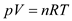Here,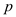is pressure,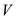is volume,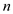is number of moles,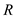is universal gas constant and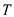is temperature. For a given mass of the gas, the number of moles constant and the value ofis constant for all the gasses. If the temperature of the gas is constant, the volume of the ideal gas is inversely proportional to the pressure on the gas. So, the plot, the pressure verses volume of the gas is a straight line with negative slope. In the above figure, the plot pressure verses volume of the gas is not a straight line, so the above plot pressure verses volume is not for the ideal gas.

The first law of thermodynamics is essentially the principle of conservation of energy, which states that in all transformations, the total heat energy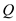supplied must be balanced by external work done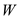plus the increase in internal energy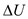of the system and expressed as,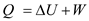Rewrite the equation for work done.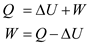Substitute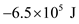for, and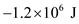for.Therefore, the work done by the person in the match is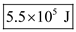.

A thermodynamics process is a change in the state of the system. The processes are classified as reversible and irreversible. Reversible process is the process in which the system could be brought back to its initial state along the same path. Every intermediate state along the path in reversible process is under equilibrium state. On a p-V diagram, the state of the system can be represented by coordinates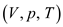. Irreversible process can be determined by knowing initial and final states and it is independent of path. Reversible process is given by very intermediate state and it should be in equilibrium which means it is path dependent. Thus, the reversible process is represented on p-V diagram when path is known. Therefore, the correct option is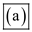.